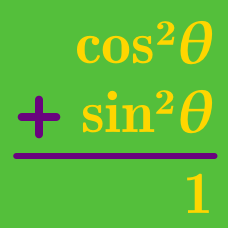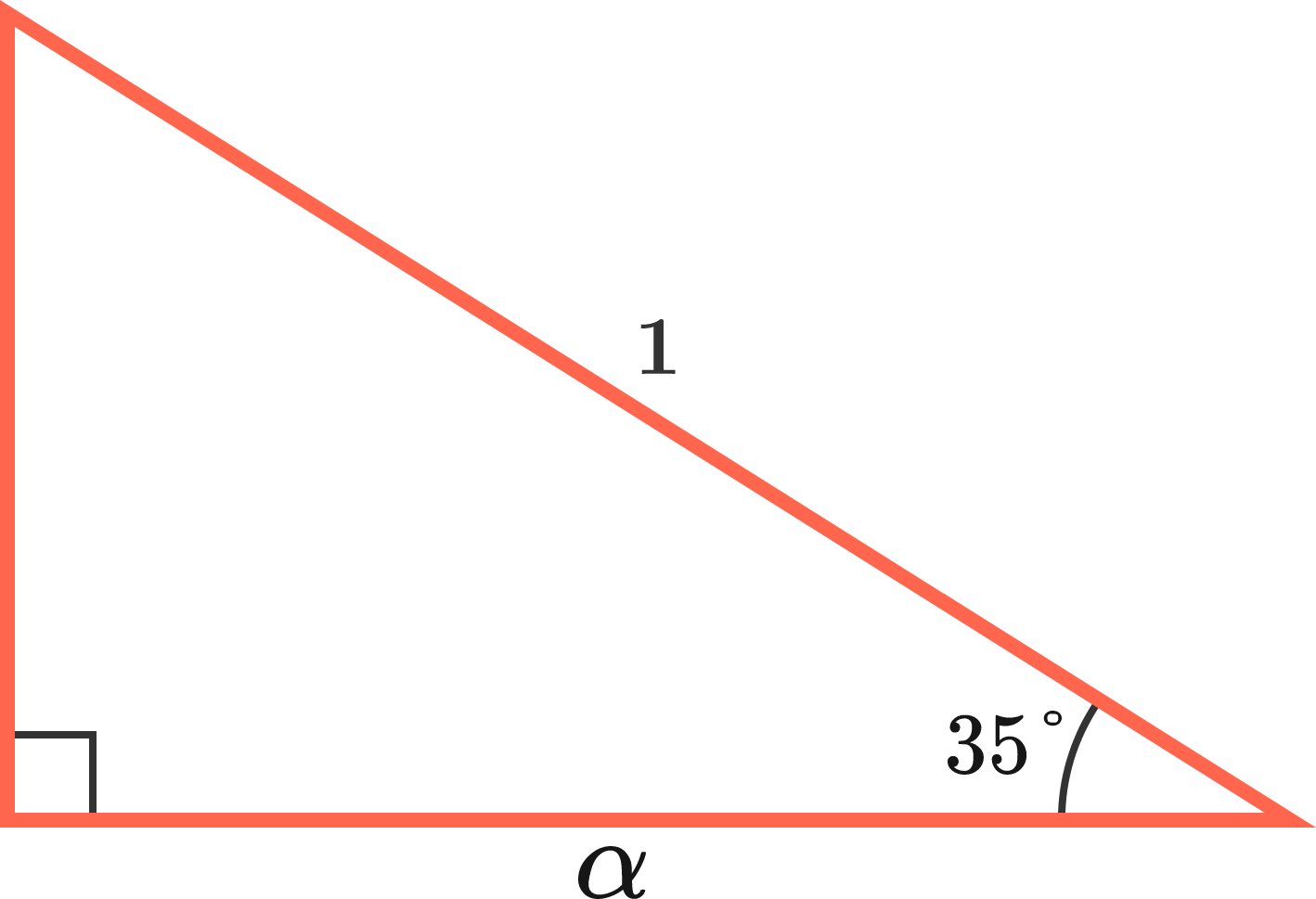Geometry

# Pythagorean Identities: Level 3 ChallengesGiven that $\cos{35°}=\alpha$, express $\sin{2015^\circ}$ in terms of $\alpha$.

This problem is part of the set 2015 Countdown Problems.

$\dfrac1{\sin^2{\theta}}-\dfrac1{\cos^2{\theta}}-\dfrac1{\tan^2{\theta}}-\dfrac1{\cot^2{\theta}}-\dfrac1{\sec^2{\theta}}-\dfrac1{\csc^2{\theta}}=-3$

Find the number of solutions of $\theta$ in the interval $(0,2\pi)$ that satisfy the equation above.

$\large 4\sin^2 (x) + \tan^2(x) + \cot^2(x) + \csc^2(x) = 6$

Find the number of solutions of $x$ in the interval $[0,2\pi]$ that satisfy the equation above.

For real number $\theta$ satisfying $1 + 2 \sin^2{\theta} = 75 \, \cos^3{\theta}$, what is the value of $3 + 4 \tan^4{\theta}$?

As $x$ ranges over all real values, what is the range of

$A=\sin^4x+\cos^2x?$

×